# MCQ in AC Circuits Part 2 | REE Board Exam

(Last Updated On: November 13, 2020)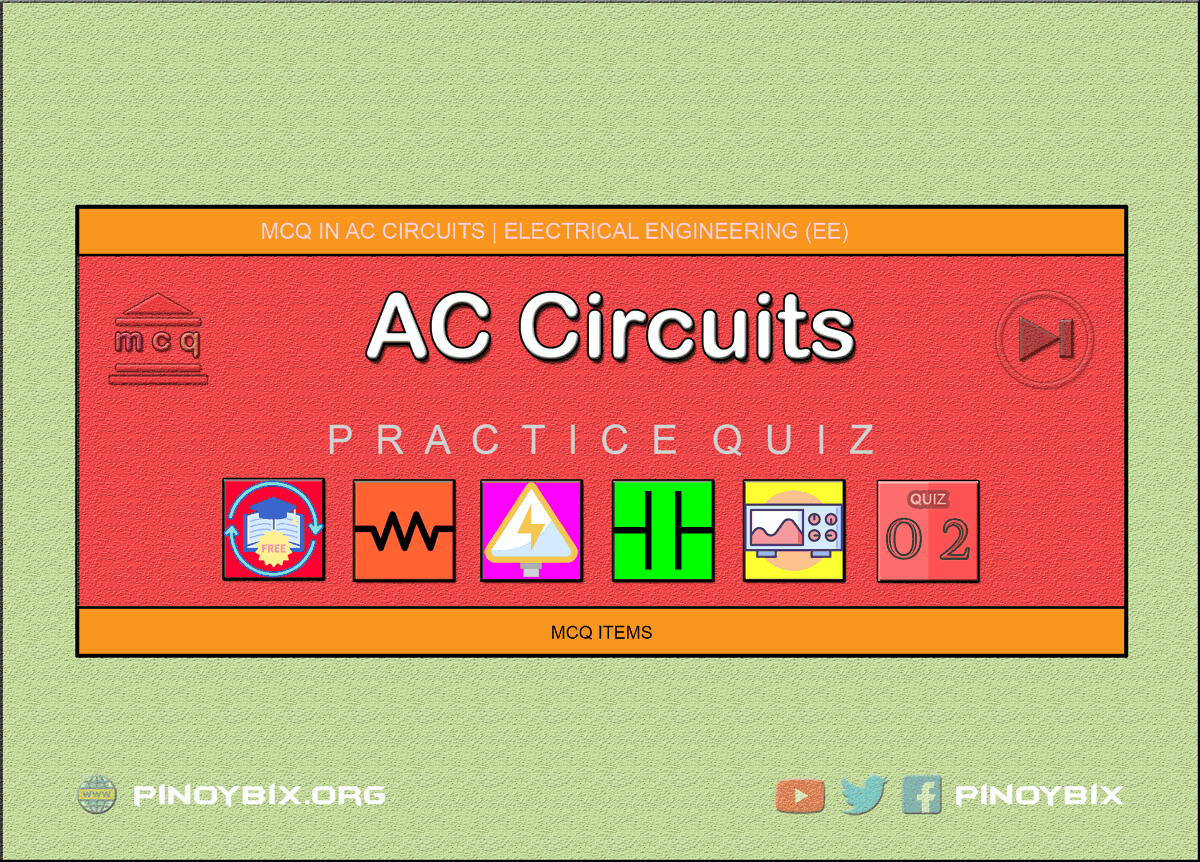This is the Multiple Choice Questions Part 2 of the Series in AC Circuits as one of the Electrical Engineering topic. In Preparation for the REE Board Exam make sure to expose yourself and familiarize in each and every questions compiled here taken from various sources including but not limited to past Board Exam Questions in Electrical Engineering field, Electrical Engineering Books, Journals and other Electrical Engineering References.

#### Continue Practice Exam Test Questions Part 2 of the Series

SINUSOIDAL VOLTAGE AND CURRENT

Choose the letter of the best answer in each questions.

51. If emf in a circuit is given by e = 100 sin 628t, the maximum value of voltage and frequency are

A. 100 V, 50 Hz

B. 50√2 V, 50 Hz

C. 100 V, 100 Hz

D. 50√2 V, 100 Hz

Solution:

52. When the sole purpose of an alternating current is to produce heat, the selection of conductor is based on

A. average value of current

B. peak value of current

C. rms value of current

D. any of the above

Solution:

53. The form factor of dc supply voltage is always

A. infinite

B. zero

C. 0.5

D. unity

Solution:

54. The frequency of a sinusoidal signal shown in figure is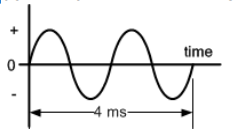A. 500 Hz

B. 1 kHz

C. 25 kHz

D. 500 kHz

Solution:

55. The period of the voltage 2 cos 4500πt + 7 sin 7500πt is

A. 2.51 s

B. 2.51 ns

C. 2.51 ms

D. 2.51 μs

Solution:

56. The a.c. system is preferred to d.c. system because ______

A. a.c. voltages can easily be changed in magnitude

B. d.c. motors do not have fine speed control

C. high-voltage a.c. transmissions is less efficient

D. d.c. voltage cannot be used for domestic appliances

Solution:

57. In a.c. system, we generate sine wave form because ______

A. it can be easily drawn

B. it produces lest disturbance in electrical circuits

C. it is nature’s standard

D. other waves cannot be produced easily

Solution:

58. ______ will work only on d.c. supply.

A. Electric lamp

B. Refrigerator

C. Heater

D. Electroplating

Solution:

59. ______ will produce a.c. voltage.

A. Friction

B. Photoelectric effect

C. Thermal energy

D. Crystal

Solution:

60. In Fig. 1.1, the component of flux that will contribute to e.m.f. in the coil is ______.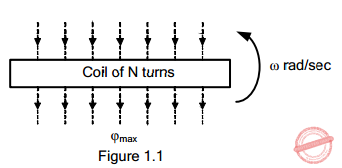A. ϕmax sin ωt

B. ϕmax cos ωt

C. ϕmax tan ωt

D. ϕmax cot ωt

Solution:

61. In Fig. 1.1, the maximum e.m.f. induced in the coil is ____­­__.A. N ϕmax

B. ω ϕmax

C. N ϕmax sin wt

D. ωN ϕmax

Solution:

62. A coil is rotating in the uniform field of an 8-pole generator. In one revolution of the coil, the number of cycles generated by the voltage is ______.

A. one

B. two

C. four

D. eight

Solution:

63. An alternating voltage is given by v = 20 sin 157t. The frequency of the alternating voltage is ______.

A. 50 Hz

B. 25 Hz

C. 100 Hz

D. 75 Hz

Solution:

64. An alternating current is given by i = 10 sin 314t. The time taken to generate two cycles of current is ______.

A. 0.02 second

B. 0.01 second

C. 0.04 second

D. 0.05 second

Solution:

65. An alternating voltage is given by v = 30 sin 314t. The time taken by the voltage to reach -30 V for the first time is ______.

A. 0.02 second

B. 0.1 second

C. 0.03 second

D. 0.015 second

Solution:

66. A sine wave has a maximum value of 20 V. Its value at 135° is _____.

A. 10 V

B. 14.14 V

C. 15 V

D. 5 V

Solution:

67. A sinusoidal current has a magnitude of 3 A at 120°. Its maximum value will be ______.

A. √3 A

B. √3/2 A

C. 2√3 A

D. 6 A

Solution:

68. An alternating current given by i = 10 sin 314t. Measuring time from t = 0, the time taken by the current to reach +10 A for the second time is ______.

A. 0.05 second

B. 0.1 second

C. 0.025 second

D. 0.02 second

Solution:

69. An a.c. generator having 10 poles and running at 600 r.p.m. will generate an alternating voltage of frequency _______

A. 25 Hz

B. 100 Hz

C. 50 Hz

D. 200 Hz

Solution:

70. We have assigned a frequency of 50 Hz to power system because it ______

A. can easily be obtained

B. gives best result when used for operating both lights and machinery

D. none of the above

Solution:

71. An alternating voltage is given by v = 100 sin 314t volts. Its average value will be ______.

A. 70.7 V

B. 50 V

C. 63.7 V

D. 100 V

Solution:

72. An alternating current whose average value is 1 A will produce ____ 1 A d.c. under similar conditions.

A. less heat than

B. more heat than

C. the same heat as

D. none of these

Solution:

73. A sinusoidal alternating current has a maximum value of Im. Its average value will be ______.

A. Im

B. Im/2π

C. 2Im

D. none of these

Solution:

74. The area of a sinusoidal wave over a half-cycle is ______

A. max. value /2

B. 2 x max. value

C. max. value /π

D. max. value /2π

Solution:

75. An alternating voltage is given by v = 200 sin 314t. Its r.m.s. value will be ______

A. 100 V

B. 282.8 V

C. 141.4 V

D. 121.4 V

Solution:

76. The r.m.s. value of sinusoidally varying current is _____ that of its average value.

A. more than

B. less than

C. same as

D. none of these

Solution:

77. Alternating voltages and currents are expresses in r.m.s. values because ______

A. they can be easily determined

B. calculations become very simple

C. they give comparison with d.c.

D. none of these

Solution:

78. The average value of sin2θ over a complete cycle is ______

A. +1

B. -1

C. ½

D. zero

Solution:

79. The average value of sinθ over a complete cycle is ______.

A. zero

B. +1

C. -1

D. ½

Solution:

80. An alternating current is given by i = Im sin θ. The average value of squared wave of this current over a complete cycle is ______

A. Im/2

B. Im

C. 2Im

D. 2Im

Solution:

81. The form factor a sinusoidal wave is ______

A. 1.414

B. 1.11

C. 2

D. 1.5

Solution:

82. The filament of a vacuum tube requires 0.4 A d.c. to heat it. The r.m.s. value of a.c. required is ______.

A. 0.4 x √2

B. 0.4 /2 A

C. 0.8 /√2

D. 0.4 A

Solution:

83. A 100 V peak a.c. is as effective as ______ d.c.

A. 100 V

B. 50 V

C. 70.7 V

D. none of these

Solution:

84. The form factor of a ______ wave is 1.

A. sinusoidal

B. square

C. triangular

D. saw tooth

Solution:

85. Out of the following ______ wave is the peakiest.

A. sinusoidal

B. square

C. rectangular

D. triangular

Solution:

86. The peak factor of a sine wave form is ______.

A. 1.11

B. 1.414

C. 2

D. 1.5

Solution:

87. When a 15-V square wave is connected across a 50-V a.c. voltmeter, it will read ______.

A. 15 V

B. 15 x √2

C. 15 /√2

D. none of these

Solution:

88. The breakdown voltage of an insulation depends upon _____ value of alternating voltage.

A. average

B. r.m.s.

C. peak

D. twice the r.m.s.

Solution:

89. The peak factor of a half-wave rectified a.c. is ______.

A. 1.57

B. 2

C. 1.11

D. 1.4142

Solution:

90. The form factor of a half-wave rectified a.c. is ______ .

A. 2

B. 1.11

C. 1.414

D. 1.57

Solution:

91. When 200 V sinusoidal peak-to-peak is connected across an a.c. voltmeter, it will read ______

A. 141.4 V

B. 50 V

C. 70.7 V

D. none of these

Solution:

92. In Fig. 1.2, the wave that will produce maximum heat under the similar conditions is ______.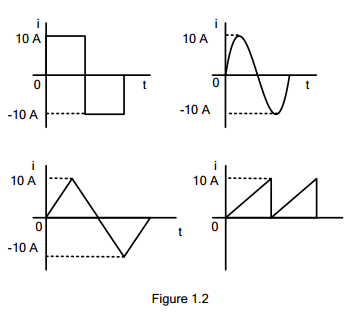A. square wave

B. sinusoidal wave

C. triangular wave

D. saw tooth wave

Solution:

93. In Fig. 1.2, ____ wave will have the highest average value.A. saw tooth

B. square

C. triangular

D. sinusoidal

Solution:

94. The average value of a sinusoidal current is 100 A. Its r.m.s value is ______.

A. 63.7 A

B. 70.7 A

C. 141.4 A

D. 111 A

Solution:

95. A current wave is given by i = 4 + 2√2 sin 3θ + 4√2 sin 5θ. The r.m.s. value of current wave is ______.

A. 10 A

B. 6 A

C. √56 A

D. 5 A

Solution:

96. In Fig. 1.3, current is given by i = Im sin θ. The voltage equation will be ______.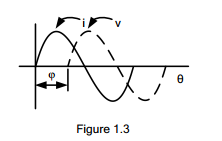A. Vm sin θ

B. Vm sin (θ + ϕ)

C. Vm sin (θ – ϕ)

D. Vm sin (θ – 2ϕ)

Solution:

97. The waveforms of voltage and current shown in Fig. 1.3 would exist in ______ circuit.A. a resistive

B. a capacitive

C. an inductive

D. none of these

Solution:

98. An alternating voltage or current is a ______.

A. scalar quantity

B. vector quantity

C. phasor

D. none of these

Solution:

99. Three parallel circuits take the following currents: i1 = 5 sin 314t, i2 = 30 sin (314t + π/2) and i3 = 25 sin (314t – π/2). The expression for the resultant current is ______.

A. 25 sin (314t + π/3)

B. 5 sin (314t + π/2)

C. 10 sin (314t – π/6)

D. 5√2 sin (314t + π/4)

Solution:

100. The sum of the following two e.m.f’s will be ______

e1 = 10 sin ωt                 e2 = 10 cos ωt

A. 10

B. 20 sin ωt

C. 14.14 cos ωt

D. 14.14 sin (ωt + π/4)

Solution:

#### Questions and Answers in AC Circuits

Following is the list of practice exam test questions in this brand new series:

MCQ in AC Circuits
PART 1: MCQ from Number 1 – 50                  Answer key: included
PART 2: MCQ from Number 51 – 100              Answer key: included
PART 3: MCQ from Number 101 – 150            Answer key: included
PART 4: MCQ from Number 151 – 200            Answer key: included
PART 5: MCQ from Number 201 – 250             Answer key: included
PART 6: MCQ from Number 251 – 300             Answer key: included
PART 7: MCQ from Number 301 – 350             Answer key: included
PART 8: MCQ from Number 351 – 400             Answer key: included
PART 9: MCQ from Number 401 – 450             Answer key: included
PART 10: MCQ from Number 451 – 500           Answer key: included

P inoyBIX educates thousands of reviewers and students a day in preparation for their board examinations. Also provides professionals with materials for their lectures and practice exams. Help me go forward with the same spirit.

“Will you subscribe today via YOUTUBE?”

Subscribe

PinoyBIX Engineering. © 2014-2020 All Rights Reserved | How to Donate? |